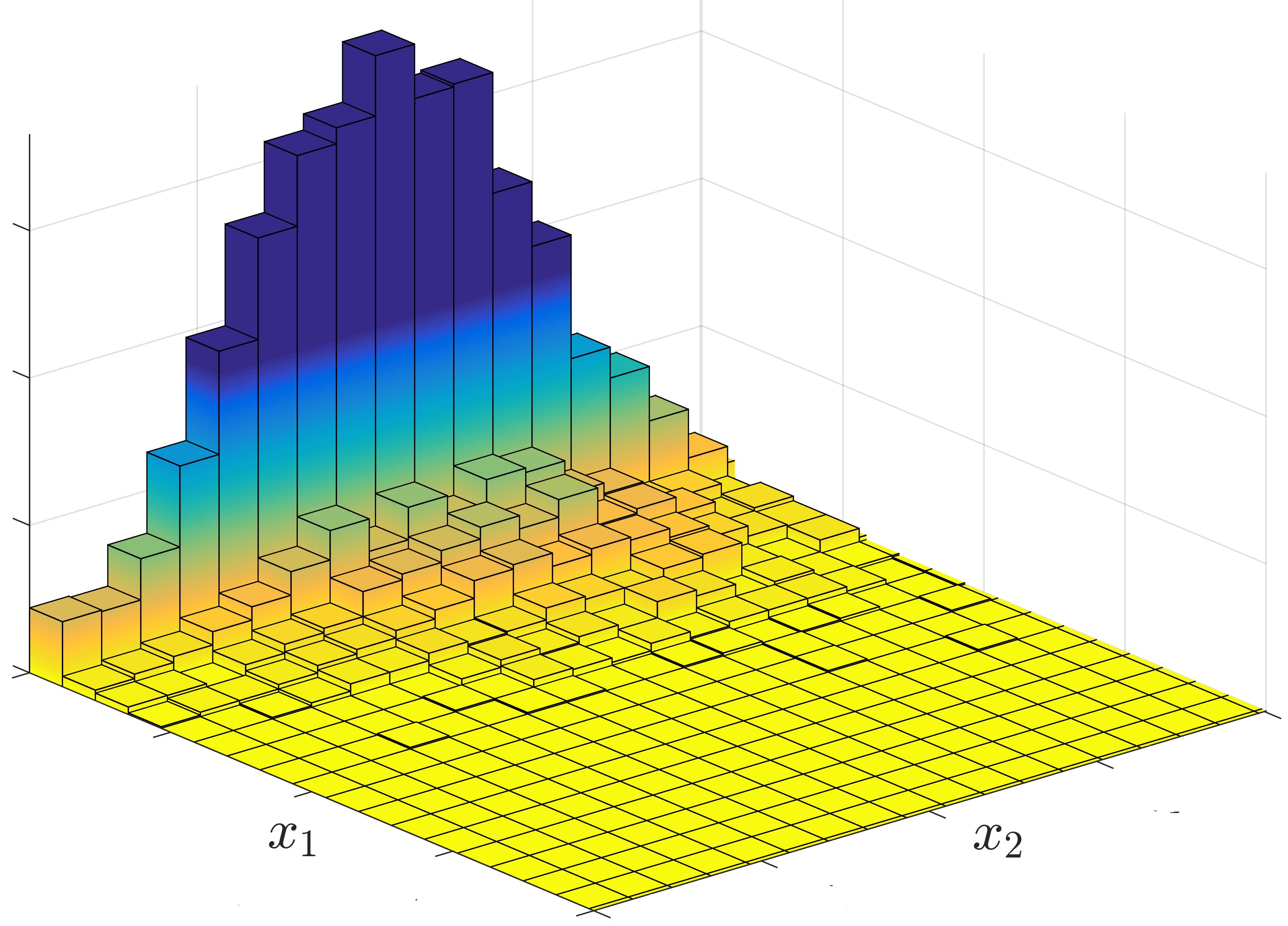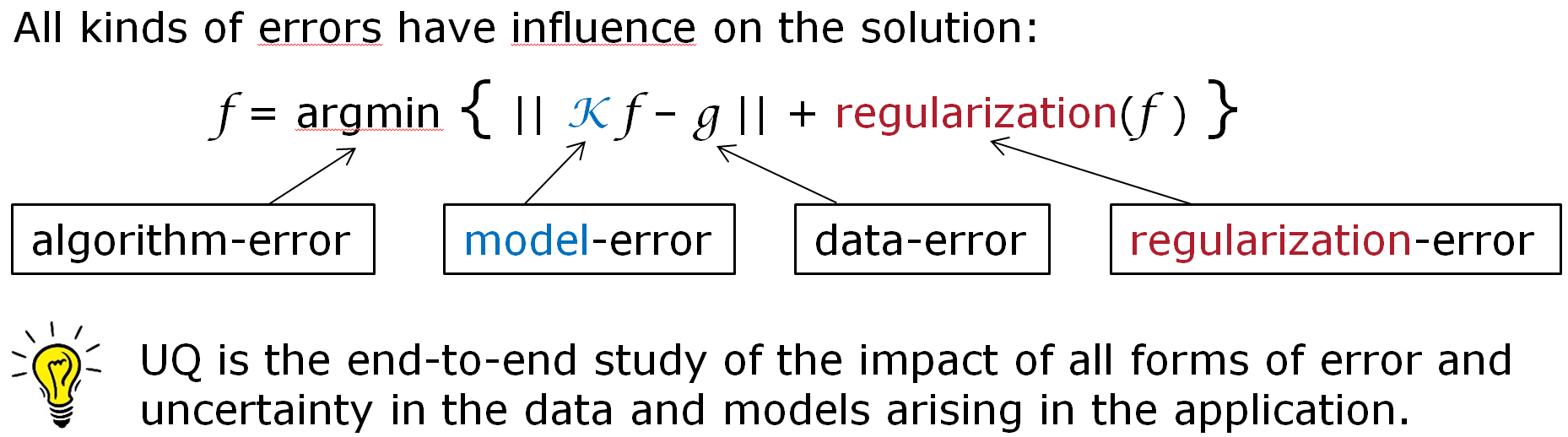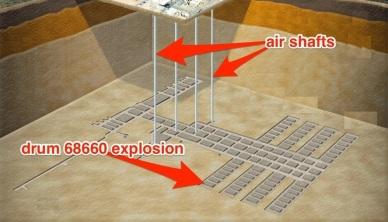# What is CUQI?

Modern reconstruction methods for inverse problems produce very sharp images with lots of details. In the present project we answer the natural question: how reliable are these details?

The key ingredient is uncertainty quantification (UQ), and our goal is to make UQ an operational tool for a range of inverse problems. Our goal is that end-users of inverse problems – in image reconstruction, tomography, etc. – will be able to quantify the reliability of their solutions without being UQ experts.

## Uncertainty Quantification (UQ)UQ is a statistical approach to analytically formulate and study the sensitivity of a solution with respect to errors in data, models, algorithms, etc. UQ is well developed for forward problems and selected inverse problems, e.g., in geoscience. But it has not yet seen a wide breakthrough as a general modeling tool for inverse problems – although it is gaining momentum.

For low-dimensional problems, UQ information can be visualized via histograms. The figure shows a 2D histogram of solutions to a simple nonnegatively-constrained least squares problem with two unknowns x1 and x2, and with Gaussian errors in the data. We see that there is a high probability that x1 = 0 and that the mean of x2 equals 1. From J. M. Bardsley and P. C. Hansen, MCMC Algorithms for Computational UQ of Nonnegativity Constrained Linear Inverse Problems.

It is an open problem how to efficiently perform, represent and visualize the UQ analysis for large-scale problems with thousands or millions of unknowns.

## Inverse Problems

Inverse problems allow us to infer about internal or hidden features from exterior and indirect measurements (see my YouTube videos); they are used daily in science, engineering and medicine. Some common examples of inverse problems are:

• X-ray computed tomography (CT) for imaging the interior of objects.

• Source reconstruction in acoustics and electromagnetics.

• Image reconstruction of noisy and corrupted images.

• Deconvolution of instrument response functions.

Mathematicians laid the foundation for inverse problems and studied their properties (e.g., existence, stability), while progress in computers and numerical algorithms made them useful in scientific and engineering applications. Often, important decisions are made on the basis of the computed solutions to inverse problems.

Faced with errors and uncertainties in an inverse problem, to what extent can we trust the solution that we compute?

The answer to this question should preferably take into account all the different  types of errors that we encounter: measurement errors in the data, model errors in forward model that links the solution to the data, errors in the regularization terms, and errors in the numerical algorithms used to compute the solution.  UQ provides a useful mechanism for doing this.### Example: Computing Certified Results via UQ

Computer simulation can be used to study groundwater transport of radionuclides from an underground deposit (see the picture) due to hydraulic conductivity. The inverse problem of determining the transport parameters for the flow, from measured data, depends on UQ to certify that the uncertainty of the transport and spread of the pollutant satisfies the given risk specifications. From O. G. Ernst and K. A. Cliffe, Uncertainty Quantification 2012: Probabilistic UQ for PDEs with Random Data: A Case Study, SIAM News, vol. 45, October 2012.

### Example: Handling Modeling Uncertainties via UQ

Consider a linear inverse problem with Gaussian errors in the measured data. We can also incorporate modeling uncertainties in the physics via a Gaussian model:

A x = b + edata + emodel ,   edata ∼ 𝒩(0,Cb) ,   emodel ∼ 𝒩(ε,CA) .

Here Cb and CA are the covariance matrices associated with errors in the data and the physical model, respectively. In the CUQI project we will develop theory and algorithms for the general non-Gaussian cases.

### Example: UQ in Image Deblurring

Image deblurring is a well-known example of an inverse problem, where we compute a sharp image from a blurred one. This example deals with rotational blur which is encountered, e.g., in astronomical imaging. The figure below illustrates how we use UQ to determine the reliability of the details in the deblurred image. The UQ visualization on the right shows the uncertainty in each pixel; white denotes high uncertainty. The center part is more reliable than the outer parts.## The CUQI Project

The ultimate goal of the CUQI project is to allow end-users of inverse problems, in science and engineering, to be able to quantify their solutions and in this way lower the risks and make more correct decisions.

The focus of the CUQI projec is on building the general theoretical and, ultimately, computational foundations to quantify how model uncertainties, measurement noise and other errors influence the solutions to inverse problems. To do this we build on the solid mathematical and statistical foundation of UQ – a modeling approach that allows us to rigorously understand, interpret and judge how the solution’s details (e.g., edges, contrasts and inclusions) are affected by various types of errors.

An obstacle is that in inverse problems we operate with a very large number of unknowns (e.g., pixels, voxels) and the computational burden can be overwhelming. Also, UQ for inverse problems raises new fundamental scientific questions that we must identify, formulate and answer properly. Hence, we need research in both theory and algorithm development.

• The theoretical outcome is a mathematical and statistical basis for UQ studies of a range of inverse problems with different priors and noise models.

• The practical outcome is a modeling platform – a computational abstraction layer aimed at non-experts.

• The overall outcome is a framework to rigorously quantify the uncertainty of the solution’s details and identify the dominating types of errors. Based on our work, UQ will become a natural part of solving inverse problems, in science and industry.

https://www.compute.dtu.dk/english/cuqi/what-is-cuqi
14 APRIL 2021